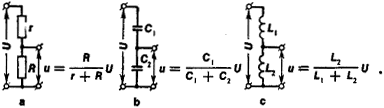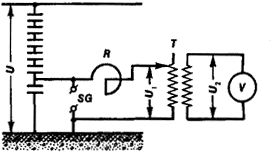voltage divider

Also found in: Dictionary, Thesaurus, Medical, Wikipedia.
Related to voltage divider: Current divider

voltage divider:

see potentiometerpotentiometer.
1 Manually adjustable, variable, electrical resistor. It has a resistance element that is attached to the circuit by three contacts, or terminals. The ends of the resistance element are attached to two input voltage conductors of the circuit, and the third
.

Voltage Divider

an electrical device for dividing a DC or AC voltage into parts. All voltage dividers consist of reactances or effective resistances.

Voltage dividers are usually used to measure voltage. At low voltages series-connected resistors (Figure l,a) are used.Figure 1. Circuits of low-voltage voltage dividers: (a) resistive, (b) capacitive, (c) inductive; (u) and (U) voltages, (r) and (R) resistances, (C1) and (C2) capacitances, (L1) and (L2) inductances

Capacitive voltage dividers with fixed or variable capacitors (Figure l,b), as well as induction voltage dividers (Figure l,c), are used for alternating currents. Capacitive voltage dividers, which consist of several series-connected high-accuracy capacitors (Figure 2), are used in electric powerFigure 2. Circuit of a high-voltage voltage divider: (U), (U1), and (U2) voltages; (SG) spark gap; (R) reactor; (T) transformer, (V) volt-meter

engineering to take off small amounts of power from a high-voltage transmission line (up to 500 kilovolts). An autotransformer is an example of an inductive voltage divider.

N. G. VOSTROKNUTOV

voltage divider

[′vōl·tij di‚vīd·ər]
(electricity)
A tapped resistor, adjustable resistor, potentiometer, or a series arrangement of two or more fixed resistors connected across a voltage source; a desired fraction of the total voltage is obtained from the intermediate tap, movable contact, or resistor junction. Also known as potential divider.
References in periodicals archive ?
To ensure consistency of the data stream the 548ADC ref+ line can be connected to the 5V supply rail rather than a voltage divider. In this way the maximum analogue output of the solar cell will not approach more than half of the 5 V maximum reference level so that if a signal greater than 128 is received by the logger computer, it will be known to have been caused by a communication synchronisation error and can be ignored.
Resistors CMP33 and CMP40 form the voltage divider. The divided down voltage can be applied to the gate terminal of the HEMT through the necessary RF isolation circuitry or an external feedback path may be created, which could lead to an oscillation condition.
8 shows the electrical circuit with the use of a high-voltage capacitive voltage divider of the type CDV-1,2, designed to form a non-standard commutation aperiodic pulse of a time shape of 250 [micro]s/5000 [micro]s of amplitude up to [+ or -]1 MV in the long air gap between the electrodes [E.sub.1] (disk) and [E.sub.2] (rod) .
An example of a voltage divider consists of two resistors in series or a potentiometer.
where [R.sub.0] is the memristance of the stored state and [R.sub.s] is the series resistance (30 K[OMEGA]) of the memristor voltage divider circuit, [V.sub.MEM] is the memristive voltage.
Traditionally voltage is measured by means of inductive instrument transformers or capacitive voltage dividers. Such transformers have been state of the art for many decades but have also a number of drawbacks.
The output signal may be specified as analog (4-20mA or 10V), voltage divider (potentiometer), digital (RS232, CanBus or DeviceNet[R]), or encoder pulse.
The two driven motor windings form a voltage divider. Knowing that the impedance variations will cause the center node to vary, our technique compares this center node to the reference voltage.
The Celesco miniature 58 mm diameter by 13 mm thick Model MTA-5 cable-extension position transducer uses a high-cycle conductive plastic potentiometer to provide a precision voltage divider position feedback signal for a full-scale measurement range of 0-5 inches.
Initially these two FETs were both connected to the control voltage through a 4:1 voltage divider that reduces the 2 V control voltage swing to about 0.5 V.
[ZD.sub.n] are Zener diodes of the D818D type; [R.sub.LV] is the resistance of the resistive voltage divider.
Measurement of peak value of voltage lightning impulse is achieved in the system using a resistive voltage divider and peak value meter.

Site: Follow: Share:
Open / Close